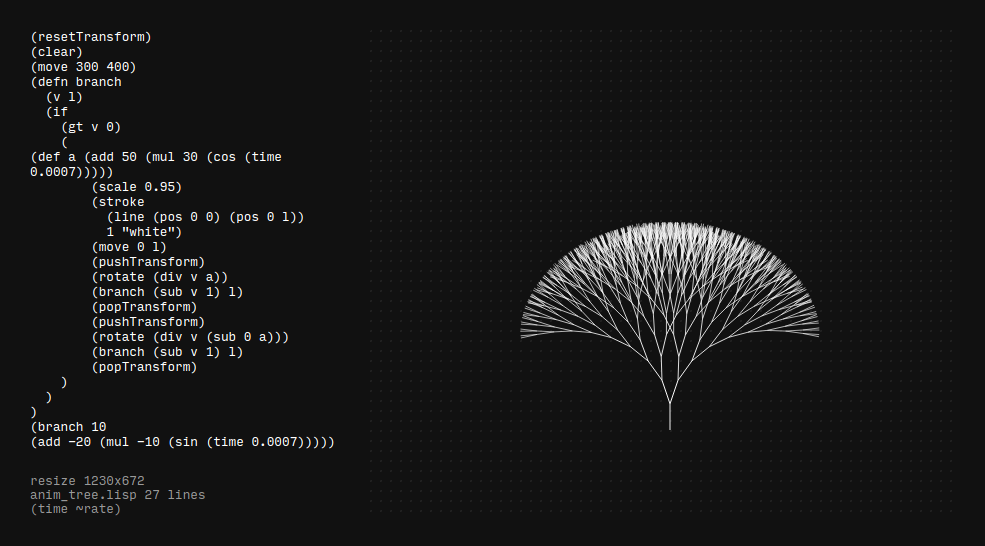## ronin experiment 6 - animated tree

I used the brand new and really awesome affine transformation features to create an anmation in RoninThis is the code i used for the animation which is based on the "transform" example

``````(resetTransform)
(clear)
(move 300 400)
(defn branch
(v l)
(if
(gt v 0)
(
(def a (add 50 (mul 30 (cos (time 0.0007)))))
(scale 0.95)
(stroke
(line (pos 0 0) (pos 0 l))
1 "white")
(move 0 l)
(pushTransform)
(rotate (div v a))
(branch (sub v 1) l)
(popTransform)
(pushTransform)
(rotate (div v (sub 0 a)))
(branch (sub v 1) l)
(popTransform)
)
)
)
(branch 10
(add -20 (mul -10 (sin (time 0.0007)))))
``````